﻿ 船舶电力推进系统异步电机直接转矩控制技术
 舰船科学技术2022, Vol. 44Issue (9): 98-101    DOI: 10.3404/j.issn.1672-7649.2022.09.020PDF

1. 郑州市机器人与智能装备工程研究中心，河南 郑州 451460;
2. 郑州市轨道交通智能检测重点实验室，河南 郑州 451460

Direct torque control technology of asynchronous motor in ship electric propulsion system
WANG Xi-yan1, CHEN Qing-hua1, LI Zheng-hui2
1. Zhengzhou Research Center of Robot and Intelligent Equipment Engineering Technology, Zhengzhou 451460, China;
2. Zhengzhou Key Laboratory of Intelligent Detection of Rail Transit, Zhengzhou 451460, China
Abstract: The main structure of ship electric propulsion system is studied, the ship propulsion system model is constructed, and the relationship between ship propulsion motor output power and ocean wave is analyzed. The control technology of marine asynchronous motor is studied. Based on the characteristics of marine asynchronous motor, the dynamic model of marine asynchronous motor and the direct torque control system of marine motor are constructed.
Key words: ship electric propulsion system     asynchronous motor     control system
0 引　言

1 船舶电力推进系统 1.1 船舶电力推进系统结构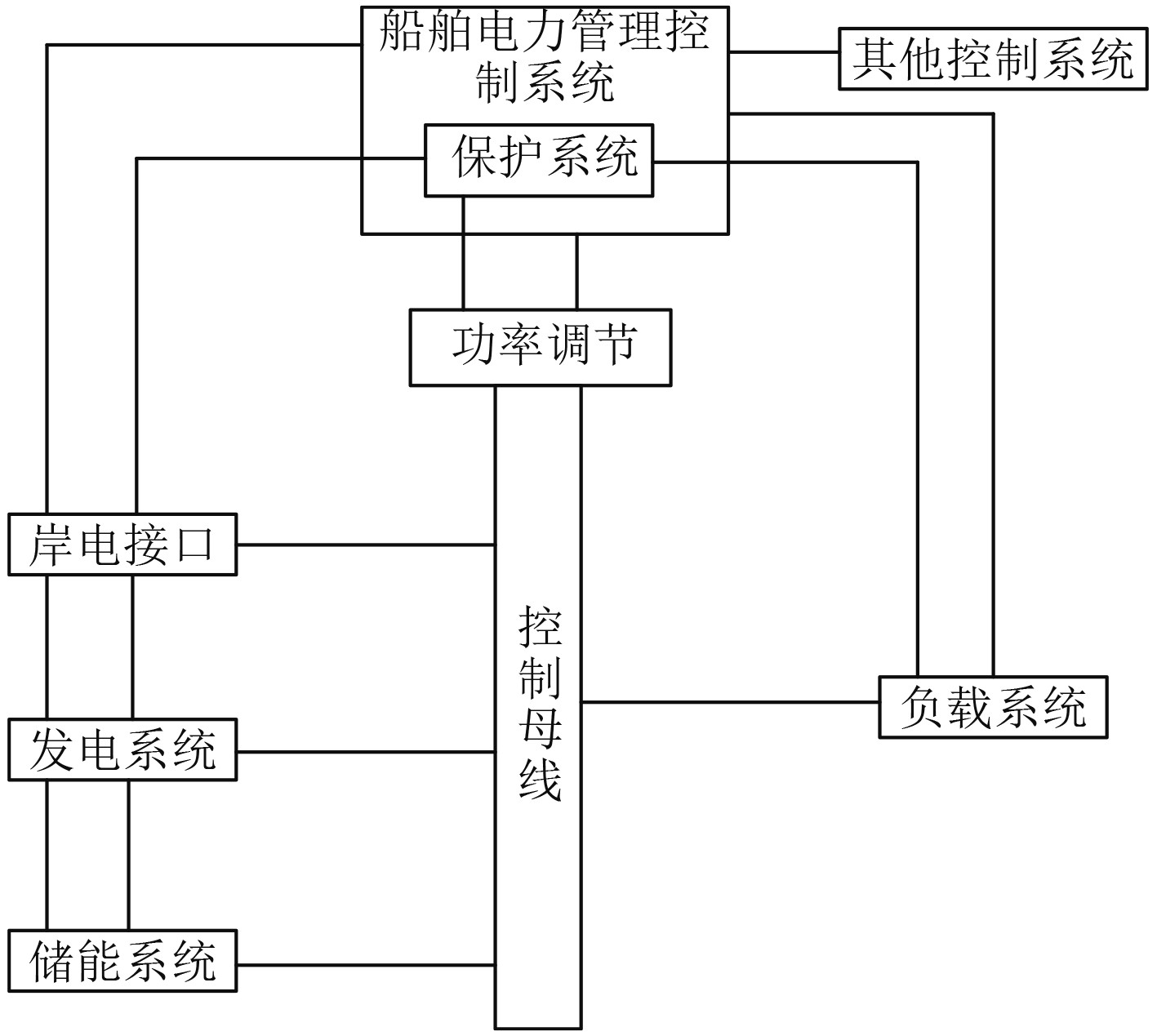图 1 船舶推进系统结构 Fig. 1 Ship propulsion system structure

1.2 船舶推进系统建模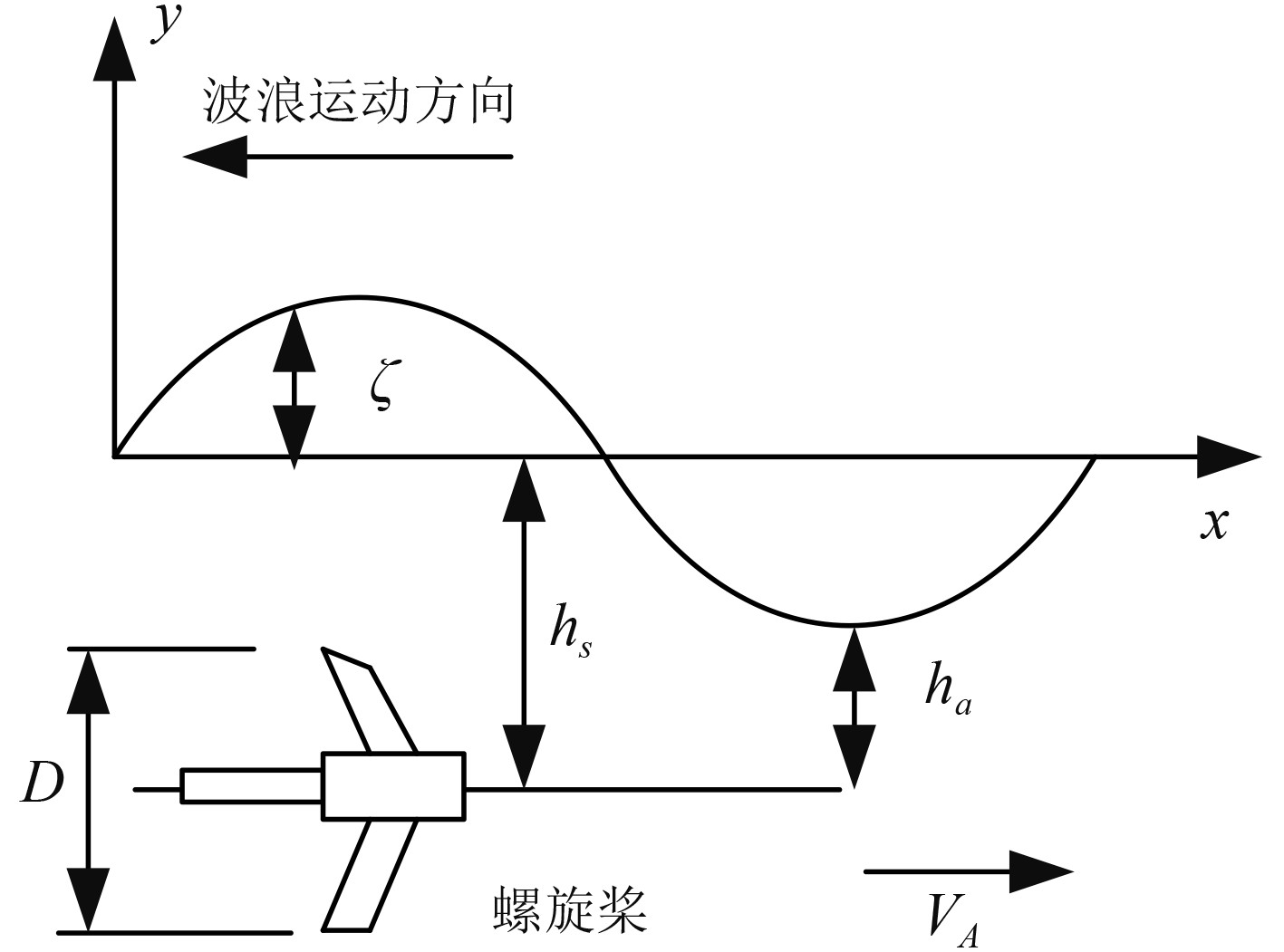图 2 波浪中螺旋桨运动模型 Fig. 2 Propeller motion model in waves
 $\zeta = a\sin \left( {Kx - \sigma t} \right)\text{。}$ (1.1)

 $\varphi = \frac{{ag}}{\sigma }{e^K}\cos \left( {Kx - \sigma t} \right)\text{。}$ (1.2)

 ${v_x} = - a\sigma {e^K}\sin \left( {Kx - \sigma t} \right)\text{。}$ (1.3)

 $V_A' = {V_A} - a\sigma {e^K}\sin \left( {Kx - \sigma t} \right)\text{。}$ (1.4)

 ${J_P} = \frac{{V_A'}}{{n{D_P}}}\text{。}$ (1.5)

 $h = {h_s} + \zeta \text{。}$ (1.6)

 $\left\{ {\begin{array}{*{20}{l}} {{X_P} = \left( {1 - {t_p}} \right)T} ，\\ {T = \rho {n^2}D_p^4{K_T}\left( {{J_P},h/D} \right)}，\\ {M = \rho {n^2}D_p^5{K_M}\left( {{J_p},h/D} \right)}。\end{array}} \right.\text{}$ (1.7)

 ${J_p} = \left( {1 - {w_p}} \right){V_A}/n{D_p}\text{。}$ (1.8)

 ${R_N} = \frac{{{b_{0.75R}}\sqrt {V_A^2 + {{\left( {0.75\text{π} n{D_p}} \right)}^2}} }}{{{v_m}}} \text{。}$ (1.9)

 ${P_{em}} = p\frac{{{L_m}}}{{{L_r}}} \varOmega {\psi _{rd}}{i_{sq}}\text{。}$ (1.10)

 ${P_m} = 2\text{ π} nM = 2\text{ π} {K_M}\rho {D^5}{n^3}\text{。}$ (1.11)

 $n = \sqrt{{\frac{{{P_m}}}{{2\text{ π} {{{K}}_M}\rho {D^5}}}}}\text{。}$ (1.12)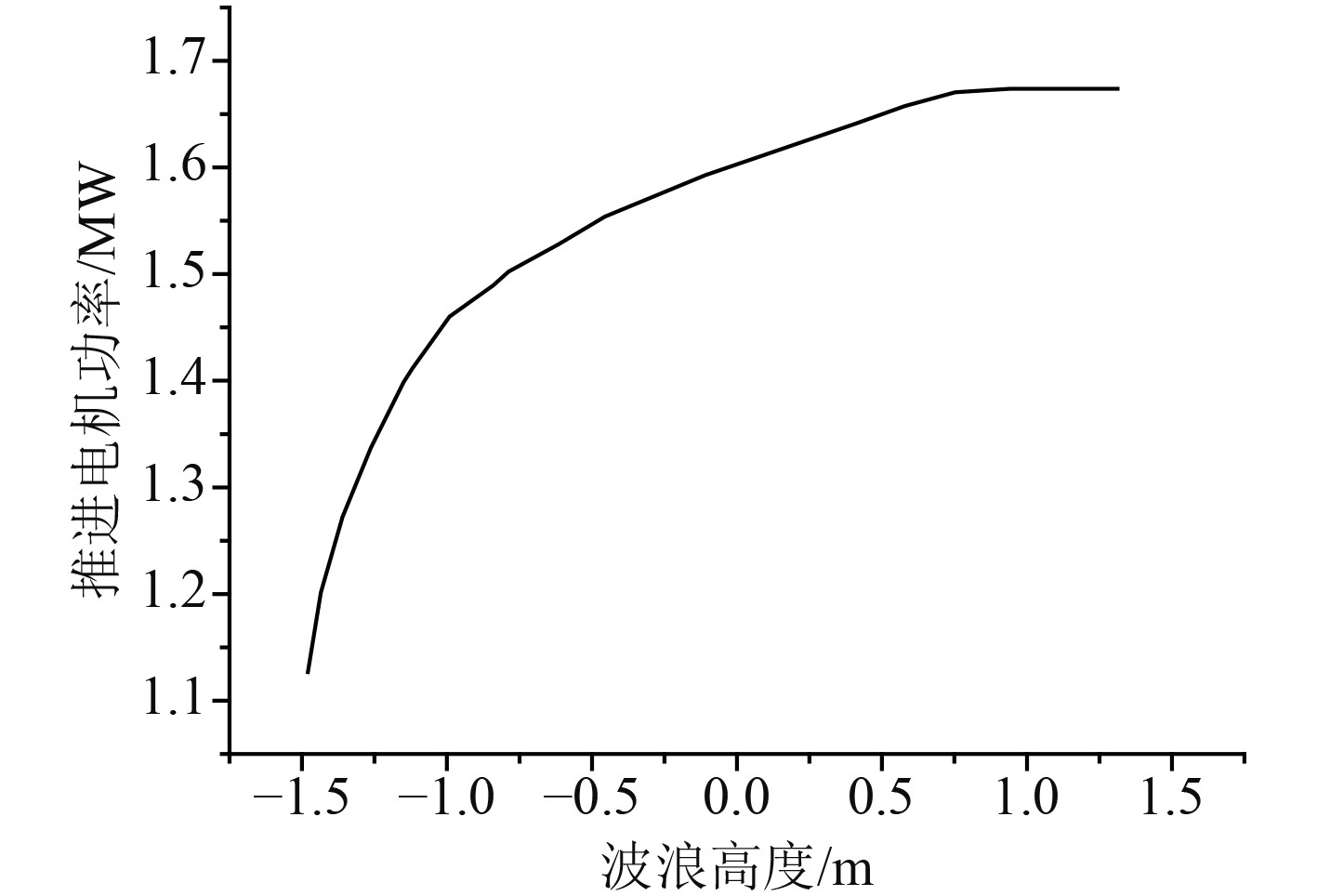图 3 船舶推进电机输出功率和海浪高度之间的关系 Fig. 3 Relationship between output power of marine propulsion motor and sea wave height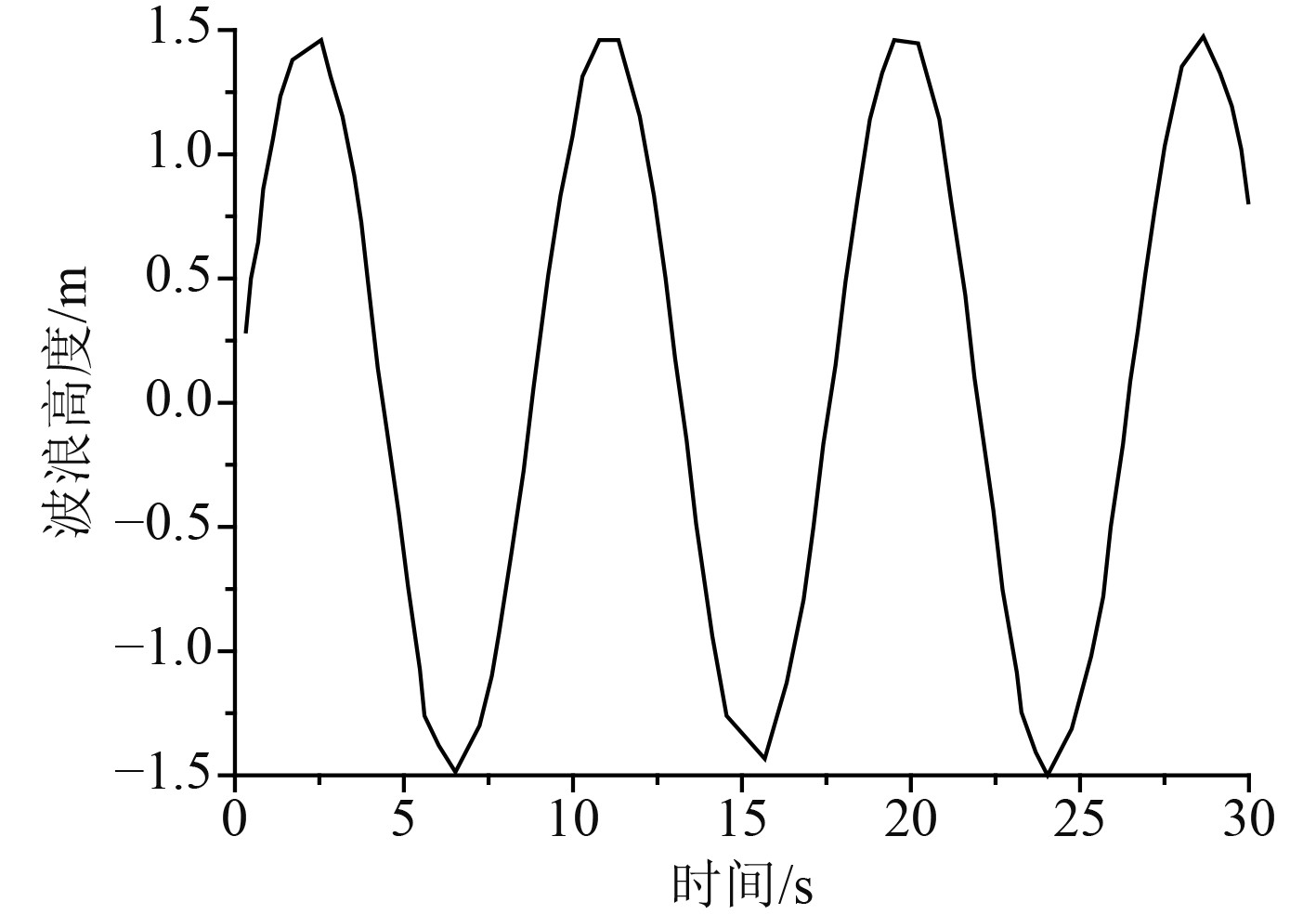图 4 海面波浪高度 Fig. 4 Sea wave height
2 船舶异步电机控制技术 2.1 异步电机数学模型

 $\left\{ {\begin{array}{*{20}{l}} {{u_A} = {i_A}{R_s} + \dfrac{{{\rm{d}}{\psi _A}}}{{{\rm{d}}t}}} ，\\ {{u_B} = {i_B}{R_s} + \dfrac{{{\rm{d}}{\psi _B}}}{{{\rm{d}}t}}}，\\ {{u_C} = {i_C}{R_s} + \dfrac{{{\rm{d}}{\psi _C}}}{{{\rm{d}}t}}} 。\end{array}} \right.\text{}$ (2.1)

 $\left\{ {\begin{array}{*{20}{l}} &{{u_a} = {i_a}{R_r} + \dfrac{{{\rm{d}}{\psi _a}}}{{{\rm{d}}t}}}，\\ &{{u_b} = {i_b}{R_r} + \dfrac{{{\rm{d}}{\psi _b}}}{{{\rm{d}}t}}}，\\ &{{u_c} = {i_c}{R_r} + \dfrac{{{\rm{d}}{\psi _C}}}{{{\rm{d}}t}}}。\end{array}} \right.\text{}$ (2.2)

 $u = Ri + P\psi \text{。}$ (2.3)

 ${T_e} = {T_l} + \frac{J}{{{n_p}}}\frac{{{\rm{d}}\omega }}{{{\rm{d}}t}}\text{。}$ (2.4)

2.2 异步电机直接转矩控制技术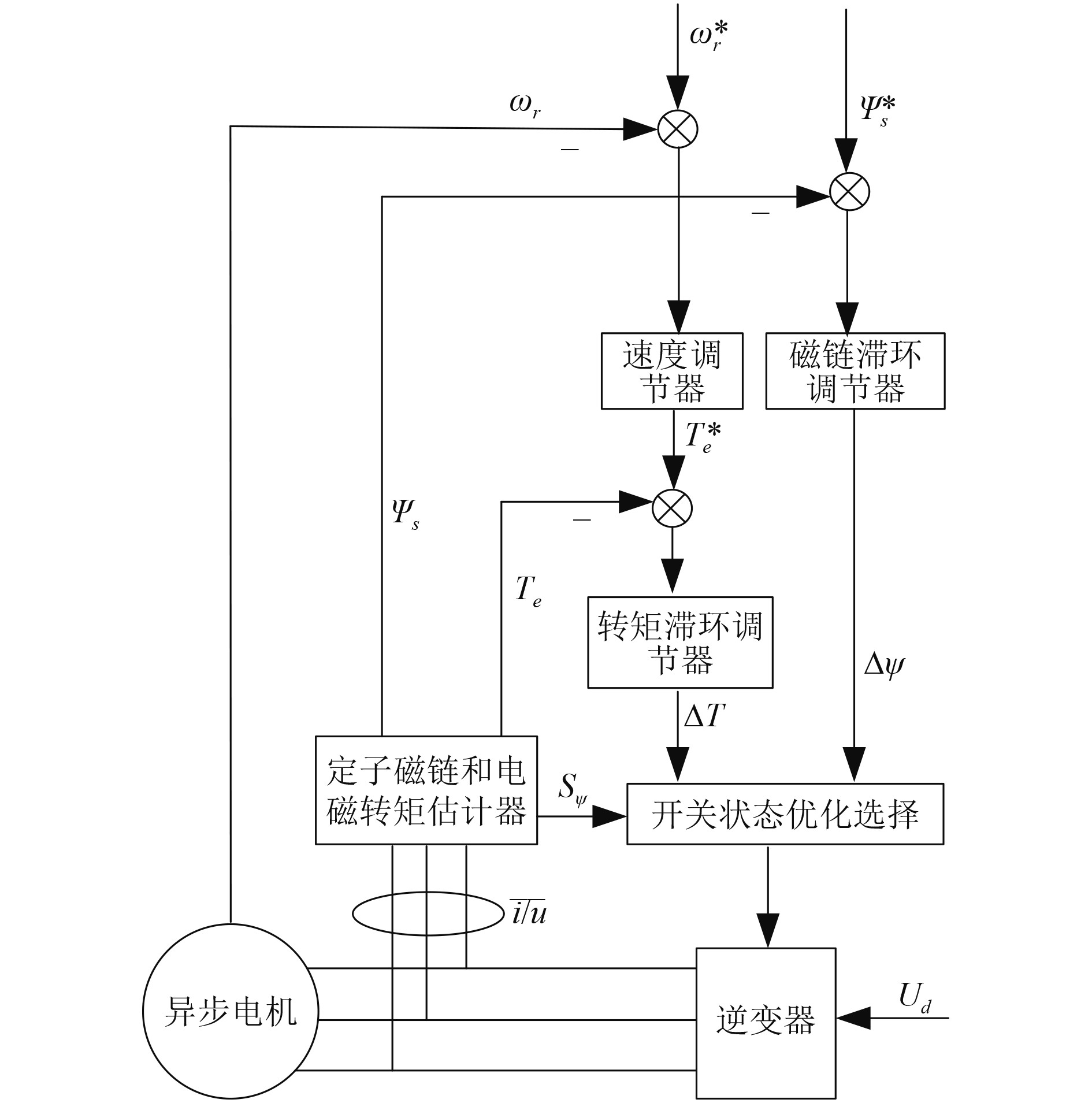图 5 异步电机直接转矩控制系统 Fig. 5 Direct torque control system of asynchronous motor

3 结　语

  纪丹. 高压整流技术在舰船电力推进系统中的应用[J]. 舰船科学技术, 2021, 43(20): 97-99.  张一驰. 基于矢量控制技术的舰船电力推进系统电机建模与仿真[J]. 舰船科学技术, 2019, 41(2): 109-111.  宋艳琼, 陈辉, 高海波. 基于直接转矩控制技术的电力推进船Saber仿真[J]. 钦州学院学报, 2016, 31(4): 6-9.  彭婧. 舰船电力推进系统工作性能的仿真分析[J]. 舰船科学技术, 2021, 43(24): 64-66.  王孟莲, 沈枫. 舰船电力推进系统故障仿真软件关键技术研究[J]. 交通信息与安全, 2010, 28(6): 5-7.  张玉婷, 卢青波. 舰船电力推进系统建模与稳定性研究[J]. 舰船科学技术, 2021, 43(12): 82-84.  冯成. 舰船电力推进系统数字化开发环境浅析[J]. 船电技术, 2009, 29(7): 60-63.  朱炜. 浅谈舰船综合电力推进系统及其相关技术[J]. 船电技术, 2014, 34(12): 14-18.  韩旗. 区域配电网络在舰船电力推进系统中应用与发展[J]. 船电技术, 2011, 31(2): 33-36.  宋文祥, 乐胜康, 吴晓新等. 一种改进的异步电机模型预测直接转矩控制方法[J]. 上海大学学报, 2018, 24(6): 861-876.  王宇, 邓智泉, 王晓琳. 无轴承异步电机的直接转矩控制技术研究[J]. 中国电机工程学报, 2008(21): 80-84.  党建军, 丁华伟. 直接转矩控制技术在三相异步电机控制中的应用研究[J]. 煤矿机械, 2012, 33(4): 198-199.